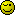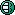# Generating Numbers Using Programming language

I am trying to write a code that will sort numbers from 1-39.

I want the results to generate 5 numbers.

They numbers should not repeat and the order matters. For example if i have {1,2,3,4,5} I cannot have (5,4,3,2,1). Is there anyone that can help with this code?

Language does not matter.

What have you tried so far? Have you thought through the logic of how you think this ought to work and perhaps generated some psuedo-code?

This is in C++. Would like to generate all the numbers that is possible.But currently is generating 1 set. Can this be converted into javascript>?

``````#include <iostream>
#include <stdlib.h>
#include <time.h>
using namespace std;

void randnum()
{
int random;
srand((unsigned int)time(0));
for(int i=1;i<=5;i++)
{
random=(rand()%39)+1;
cout<<random<<endl;
}
}

int main()
{
cout<<"Five random number is here"<<endl;
randnum();
system ("PAUSE");
return 0;
}
``````

I don’t know C++ at all, so can’t easily interpret that. If I’m understanding it correctly though, you just need to output random set of 5 numbers from a larger set that runs 1-39, and the output set needs to be presented in numerical order.

All possible numbers from 1-39. No numbers should be duplicated. For example what is not needed: {1,1,2,3,4} and also numbers in this format should not appear {1,2,3,4,5} - {5,4,3,2,1} Because it is same but its in a different order. I tried java-script but can seem to think about how to write the algorithm to solve it. Could you help?

Sounds like a task for perl.

Here’s one way:

``````const arr = [];
while(arr.length<5){
const num = Math.floor(Math.random() * (39+1));
if (arr.includes(num)) continue;
arr.push(num)
}
arr.sort((a, b) => { return a - b; });
console.log(arr);
``````

Does this do what you want?

Hi there Pullo,

I think that your example may require this modification…

``````console.log( arr.sort(  ( a, b ) => { return a - b; } ) );
``````

…to put them in numerical order.2 Likes

1 Like

I have updated my post also, to reflect your
preferred use of the arrow function.You find some strange things amusing, coothead3 Likes

Do not be alarmed, I find all strange things to be amusing.Probably unbeknown to you, I also tend to smile benignly.1 Like

It works but how do I make generate all the possible numbers? Currently is this dispaying random number. I want to display all possible numbers

You just want to log numbers 39-1?

Yes 1 to 39 numbers, Zero should not be includedThis?

``````for(let x=1; x<40; x++) console.log(x);
``````

It would be useful if you could give more thought to how you describe the problems you wish to solve. So far, I feel like there have been at least three different questions in this thread alone, all of which would necessitate a different solution.

2 Likes

Okay i want to display 5 numbers from 1 to 39. (All possible numbers). The numbers should not be duplicatedd and the order does not matter. However numbers in this format should not appear {1,2,3,4,5} - {5,4,3,2,1} becuase it the same. Also number format like this should not appear {1,1,2,3,4} . Zero Should not be included

This code works. However its just displaying 1 set of 5 numbers only. It should be displaing all possible numbers of set of 5

``````<Doctype html>
<body>
<script>
const arr = [];
while(arr.length<5){
const num = Math.floor(Math.random() * (39+1));
if (arr.includes(num)) continue;
arr.push(num)
}
arr.sort((a, b) => { return a - b; });
console.log(arr);
</script>
</body>

<html>

``````

I can’t be the only one for whom that’s not making much sense. The code shown gives you a single set of 5 numbers, which appears to be what you’re looking for. You then say you want all possible numbers from 1 to 39. Are you looking for multiple sets of 5 to achieve that, or something different?